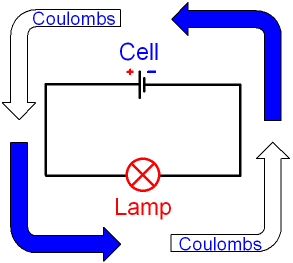gcsescience.com                                       3                                       gcsescience.com

Electricity

What is a Coulomb?

A coulomb is a unit of electrical charge.
Charge is given the symbol Q.

Electrons are tiny and have a very small charge.
In the physics of electricity, we take a
very large number of electrons as 1 unit of charge
called a coulomb.

1 coulomb = 6·2 x 1018 electrons.
This is 6·2 million million million electrons.
Such a large number of electrons
can do useful things like light a lamp.

What is Direct Current?

There are two different types of power supply.
One type supplies alternating current
and the other type supplies direct current.
A cell or a battery supplies direct current.

With direct current, electrons leave from one side of
the cell, pass through all of the components in
the circuit and return back to the other side of the cell.

The electrons are given energy by the power supply
and they then transfer this energy to all of the
components in the circuit. Electrons are not used up
by the components. The same number of electrons
leave from one side of the cell and return to the other.What do we need to know about Coulombs?

We need to know

1. The rate of coulombs flowing in the circuit.
The number of coulombs per second is called the current.

2. How much energy each coulomb has.
The number of joules per coulomb is called the voltage.

gcsescience.com      Physics Quiz      Index      Electricity Quiz      gcsescience.com